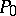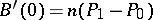# Bézier curve

Jump to: navigation, search

A parametric polynomial defined by a degree,, and a sequence of"control points" ,. The resulting curve is thenSee Fig.a1 for an example of a cubic Bézier curve. These curves are closely related to Bernstein polynomials, and are sometimes called Bernstein–Bézier curves. The major difference between Bézier curves and Bernstein polynomials is that Bézier curves are a parametric vector-valued representation based on control points, whereas Bernstein polynomials are usually thought of as a sequence of real-valued approximants to another function.Figure: b110460a

A cubic Bézier curve

Bézier curves appear in such areas as mechanical computer aided design (CAD). They are named after P. Bézier, who used a closely related representation in Rénault's UNISURF CAD system in the early 1960s (similar, unpublished, work was done by P. de Casteljau at Citroën in the late 1950s and early 1960s). The 1970s and 1980s saw a flowering of interest in Bézier curves, with many CAD systems using them, and many important developments in their theory.

The usefulness of Bézier curves resides in their many geometric and analytical properties. There are elegant and efficient algorithms for evaluation, differentiation, subdivision of the curves, and conversion to other useful representations. Moreover, Bézier curves interpolate their end-points (and), have derivatives at the end-points depending only on the first few or last few control points (e.g.,), are invariant under affine transformations (the transformed curve is identical to the curve obtained by applying the transformation to the control points, and then calculating the resulting Bézier curve), lie in the convex hull of their control points, and oscillate no more than the piecewise linear interpolant to their control points. Bézier curves also extend to surfaces (which are even more important for design purposes, cf. Bézier surface), volumes, etc. in a natural manner. There is also a rational extension of Bézier curves that allows exact representation of conic sections. These properties and algorithms make the curves popular in areas where shape is important. In addition to CAD, examples of such areas include computer animation and font design.

Bézier curves are not the only representation used in these areas. One drawback of Bézier curves is that they approximate their control points, while some designers prefer to use an interpolating representation. A larger drawback is that Bézier curves are a polynomial representation and do not have the flexibility and generality of piecewise-polynomial representations such as-spline curves (cf. also Bézier spline; Box spline). For example, Bézier curves do not have the local control property possessed by many piecewise-polynomial representations: changing one control point of a Bézier curve affects the entire curve. Using the end-point derivative property, it is possible to join Bézier curves to obtain piecewise-polynomial curves, but doing so creates additional concerns not present in the-spline representation.

Therefore, some CAD systems use Bézier curves and surfaces, some employ the-spline representation or NURBS (rational-splines), and a few use still other representations. There is actually an elegant link between-spline and Bézier representations, since Bézier curves can be thought of as-spline curves with all the-spline knots clustered at the end-points of a single interval. Because of this, many algorithms for-spline and Bézier curves and surfaces share the same general form, and many CAD systems based on-splines or NURBS contain Bézier curves and surfaces as a special case.

There are a number of references on Bézier curves and surfaces, with [a1] the most commonly used one.